Josephson Superconducting Tunneling Effect

Cheng-Chien Chen March 17, 2007

(Submitted as coursework for Applied Physics 272, Stanford University, Winter 2007)

In quantum mechanics we know that an electron can tunnel through a barrier, even if its kinetic energy is less than the barrier potential, thereby producing a tunneling current. In this report, I shall describe a different kind of tunneling in the superconductors, in which superconducting pairing between electrons is important. This kind of tunneling, predicted by B. D. Josephson in 1962 , was first confirmed experimentally in 1963 by P. W. Anderson and J. M. Rowell . Their experimental sample, a superconductor-insulator-superconductor (SIS) junction, is illustrated in Figure 1.

There are two kinds of Josephson tunneling, known as the DC and AC Josephson effects. We focus here only on the DC Josephson effect, which was the subject of the Anderson and Rowell paper. To begin with, I shall show how Anderson and Rowell measured this tunneling current by giving the (sketchy) plots of the apparatus and the data they had. After a brief review of Josephson's thoery, we will be able to understand how they can make sure at that time that what they measured was indeed the Josephson current based on a few experiment point of views, and hence ruled out the possibilities of ordinary electron tunneling.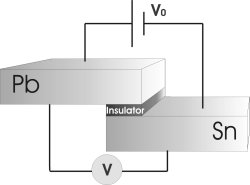Fig. 1: The SIS Josephson junction used to detect the Josephson tunneling currect. The junction has dimensions 0.025x0.065 cm2.

Measurement of the Josephson Effect

The junction illustrated in Fig. 1 consists of an insulating oxide barrier between two thin lead and tin films, both of which are approximately 2000 angstrom thick. One possible way to fabricate the insulating barrier is by exposing the connection part between the two metals to the air. Anderson and Rowell applied a known voltage difference, say V0, across the two metals (in the super-conducting state), and the voltage drop across the two metals can be determined by attaching a potentiometer across them. The way they did in 1963 was by measuring the corresponding voltage drop across a 10 ohm resistor on the loop connecting the two metals, and then the resultant Josephson tunneling current through the barrier can be known from the Ohm' law.

Their measured (tunneling) current versus (applied) voltage characteristic plot is given in Figure 2. It should be pointed out that the tunneling current is quite sensitive about the magnetic field as is shown in the figure. Even a few gauss difference can cause a lot of difference in the tunneling current (see the discussion in the Josephson's theory part). The linear part (in Figure 2) involves quasi-particle tunneling for the driven voltage is now large enough, while the current with voltage smaller than the threshold value is recognized as the Josephson tunneling current (the dashed line).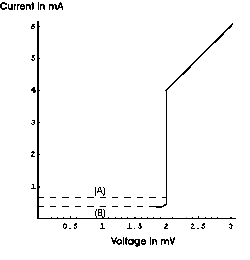Fig. 2: The Current-Voltage characteristic plot for a tin oxide-tin junction, (A) for a field of 6x10-3 gauss, and(B) for a field of 0.4 gauss.

Introduction to Josephson's Theory

In 1962, Josephson published a ground-breaking paper, which predicts a new tunneling effect in superconductors. The basic result is that when one applies a voltage drop across the SIS junction as shown in Figure 1, the current flowing through the insulating barrier Is would reads that[1,3]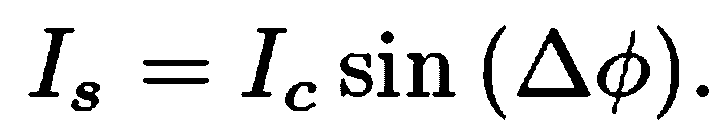where the phase difference in the sin function can be found from the following differential equation: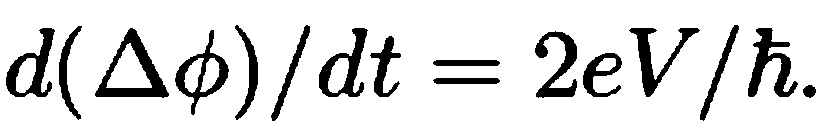Here, V is the applied voltage mentioned before, and the constants involved in the coefficient are the electron charge and Plank's constant. Therefore, if one applies an DC voltage across the junction, according to the above two equations, one can then measure an AC current through the insulating barrier. When there exists a magnetic field, the equation for the tunneling current must be modified to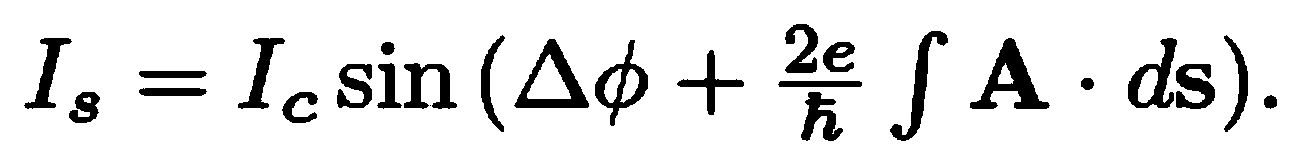where A is the corresponding vector potential of the magnetic field. Because of the appearance of the Plank’s constant in the denominator of the integral, a rapid oscillation will be resulted in even for a weak magnetic field. Actually, as suggested by Anderson, the absence of Josephson tunneling in other experiments (before theirs) may be due to the Earth’s magnetic field (about 0.5 gauss), which is already strong enough to cause rapid oscillation of the phase (and hence smears out the Josephson tunneling current.)

For future use, we address a little bit about the influence of fluctuation on the Joseph tunneling current. To see this, we rewrite the equation for tunneling current by putting an additional fluctuation: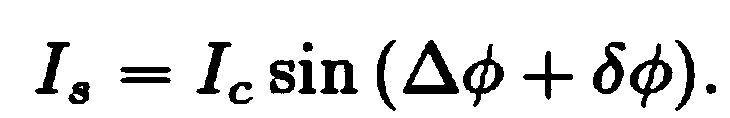Expand the above equation and notice that the average of the sine function (with the fluctuation as its argument) is zero, one then has the following equation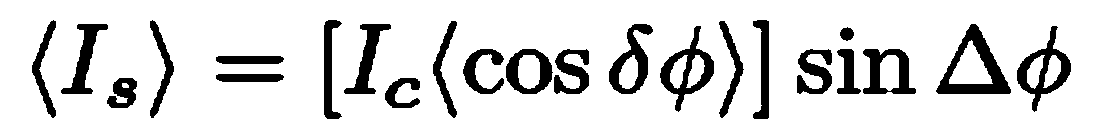therefore, the current is being reduced under fluctuation.

Some Experimental Points to Confirm the Measured Josephson Tunneling

Admitted, it is possible that what Anderson and Rowell measured at that time was just the ordinary quantum mechanical tunneling, instead of the Josephson effect; however, there were a few experiments which can further confirm that their result is indeed what Josephson predicted in his paper:

(1) As mentioned before, the effect should be very sensitive to magnetic fields. They did their experiment by blocking external magnetic fields with the aid of a mu-metal shielding, where the measured interior magnetic field is about 6x10-3 gauss. As can be seen from Figure 2, the resultant I-V characteristic plot is quite different for different magnetic fields, which is what people won't expect for an ordinary electron tunneling.

(2) The observation of this tunneling occurs only when both metals, the lead and the tin, become superconductors. As pointed out in the very beginning, the Josephson effect is a new kind of tunneling for superconductors (or more precisely, for a junction with two superconductors); therefore one would expect that no Josephson tunneling can be measured before the metals transit into the super-conducting states.

(3) They tried to continue increasing the current through the barrier, in order to burn out the junction, and found that when the junction was being burned out, the characteristic I-V curve changed drastically at the same time. Again, this can be understood by the fact that the SIS junctions are necessary to observe the Josephson effect.

Based on all the above arguments, Anderson and Rowell then made sure that they successfully observed the Josephson tunneling effect. What might concern them was that their measured tunneling current is about one order of magnitude less then the theoretical prediction. However, this is not hard to understand if one takes into account the fluctuation effect as discusses above.

© 2007 C.-C. Chen. The author grants permission to copy, distribute and display this work in unaltered form, with attribution to the author, for noncommercial purposes only. All other rights, including commercial rights, are reserved to the author.

References

 B. D. Josephson, Phys. Lett. 1, 251 (1962).

 P.W. Anderson and J. M. Rowell, Phys. Rev. Lett. 10, 230 (1963).

 M. Tinkham, Introduction to Superconductivity (McGraw Hill, 1980).